Next: References Up: Applications/New Directions Previous: Distance Problems

## Open Questions

We present some open questions related to the fixed point property in the following. Some are more precise formulations of problem 1.1 for special cases, others are intended to put methods developed for use with the fixed point property to use in other areas.

1.   For what classes of ordered sets does Xia's reduction algorithm decide if an ordered set has a fixed point property? I.e., (cf. Theorem 2.22) for what classes of ordered sets does termination of Xia's reduction algorithm with a non-empty graph imply the existence of a fixed point free order-preserving map?
2. What is the average case complexity of problem 2.3. (For average case complexity cf. .)
3.   Is it possible to decide in polynomial time whether a given ordered set is (connectedly) collapsible? Does Xia's algorithm terminate with a graph with no edges when applied to a connectedly collapsible ordered set? (There is an algorithm that decides connected collapsibility in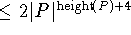comparability checks, cf. .)
4. Is there an infinitary analogue of connected collapsibility, which again implies that all fixed point sets are connected? This might also be a first step towards an analogue of acyclicity for infinite ordered sets/graphs/simplicial complexes.
5. Is it possible to decide in polynomial time whether a given ordered set is acyclic?
6.   Is the problem whether a given truncated lattice has a fixed point free order-preserving self-map NP-complete? (Section 4.8 seems to indicate that the problem is quite complex.)
7. The next problems have the same purposely vague formulations as Problem 1.1 to allow for a wide variety of possible approaches. (Naturally we can also ask the complexity question.)
1. (compare with , problem on p.233) Characterize all (finite) ordered sets P such that each order-preserving map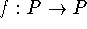has a connected nonempty set of fixed points.
2. Characterize all (finite) graphs that have the fixed clique property. (Some theorems are presented in  and in . More questions on fixed cliques appear in .)
3. Characterize all (finite) simplicial complexes that have the fixed simplex property. (Some theorems are presented in .)
4. Characterize those ordered sets without infinite chains that have the relational fixed point property (cf. section 3.5).
8.   Let P,Q,R be finite ordered sets and assume Q is dismantlable. Does the fact that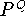is isomorphic to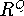imply that P is isomorphic to R? Can we use other families of retractions to arrive at results similar to Proposition 5.5?
9. Find a nondismantlable finite ordered set P such that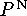(where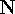carries the discrete order) has the fixed point property. This is a natural generalization of the product problem which was solved in  for finite ordered sets. It is easy to see that if P is finite and dismantlable, thenalso is dismantlable. However there are no results for infinite powers of non-dismantlable finite ordered sets. A possible first candidate would be the set P1 in  (which first appeared in ). It is connectedly collapsible, which might allow for arguments along the lines of . The fact that we have infinitely many factors adds a large degree of difficulty however.
10. Are there good estimates on how many order-preserving self maps of an ordered set, i.e., what fraction of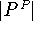, must have a fixed point (cf. )?
11. Are the sets of fixed points of self maps of Z-acyclic ordered sets always connected? (For Q-acyclic ordered sets the answer is negative as example 2.2 in  shows.)
12.   What graph theoretical analogues are there of the Li-Milner theorem?
13. Is the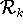-core of an infinite set unique if it exists? Is the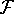-core of an infinite set unique if it exists? (Clearly there are nontrivial-cores: The rational numbers with the natural order are an-core.) Is the core in the sense of Li and Milner unique if it exists (cf. Question 1 in , p.353)?
14. Is it possible to prove uniqueness results for ``cores with respect to dismantlability via escamotable points" similar to Theorem 3.14?
15.   Is it possible to prove analytical results that use the conditional completeness of the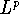-spaces resp. the lattice structure of spaces of continuous functions? (E.g. to obtain a starting point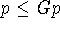one could look for a function f such that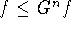and use the supremum of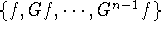as p. This idea is exhibited in , Corollary 2.)
16. (This question is due to B. Larose,  and is mentioned also at the end of . Common fixed points were for example also considered on topological spaces for continuous maps in , resp. for families of commuting order-preserving maps in .) Under what conditions on the ordered set P do all automorphisms of P have a common fixed point? Is the fixed point property or any of the many sufficient conditions for the fixed point property also sufficient for this property?

Acknowledgements (this mathematician's apology):

Much of this survey stems from preprints that were made available to me by various researchers and from private communications. Among the individuals that I wish to thank are K. Baclawski (for a preprint of ), D. Duffus (for informing me about the work of Goddard and Williamson and a preprint of ), J.D. Farley (for the argument after the introduction of Lefschetz numbers, a copy of  and for pointing out ), T. Goddard (for letting me use proof 1 of Theorem 2.14), S. Hazan (for a copy of  and an early version of ), S. Heikkilä (for a large number of reprints on nonlinear analysis), S. Homer (for a preprint of ), T. McKee and E. Prisner (for a preprint of  and the permission to include a translation to Z-homology), I. Rival (for the invitation to write this, patience as I was writing it and much general encouragement), M. Roddy (for the very inspiring collaboration on finding an example of an ordered set with the fixed point property for which Xia leaves some edges), A. Rutkowski (for a preprint of  and delightful collaboration on ), G. Sabidussi (for references on the fixed clique property and the final coordinates of ), R. Stanley (for pointing out  and ), J. Wang (for a preprint of ), plus an unknown referee (for the argument on triangulations of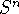), but most of all my wife and children (for continuing to put up with me). The strong influence of very recent results shows how fertile this field and its connections to analysis, algebraic topology, complexity theory, graph theory are (in fact the paper  as well as the notion of connected collapsibility materialized during the writing of this manuscript). The style of presentation definitely is my own as I frequently changed the original presentation to fit the most difficult of conditions: It had to be simple enough so even I could understand it. The algebraic topology chapter is definitely too brief and too dense, yet I hope it will do for other researchers who currently don't use algebraic topology the same that it did for me: Provide a start into using this powerful theory. Likewise the section on applications to analysis is too short, but I hope that the mentioning of this body of work will direct more attention in this direction. (As seen in question 15 my ideas in this direction are quite trivial and possibly impractical so far.) I apologize for any over-simplifications, missing references and unclear spots that may remain and I hope that any remaining errors in the manuscript are not mathematically significant.Next: References Up: Applications/New Directions Previous: Distance Problems

Bernd.S.W.Schroder